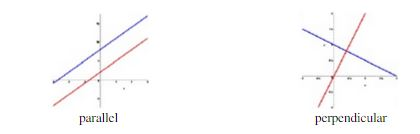Ontario Tech acknowledges the lands and people of the Mississaugas of Scugog Island First Nation.

We are thankful to be welcome on these lands in friendship. The lands we are situated on are covered by the Williams Treaties and are the traditional territory of the Mississaugas, a branch of the greater Anishinaabeg Nation, including Algonquin, Ojibway, Odawa and Pottawatomi. These lands remain home to many Indigenous nations and peoples.

We acknowledge this land out of respect for the Indigenous nations who have cared for Turtle Island, also called North America, from before the arrival of settler peoples until this day. Most importantly, we acknowledge that the history of these lands has been tainted by poor treatment and a lack of friendship with the First Nations who call them home.

This history is something we are all affected by because we are all treaty people in Canada. We all have a shared history to reflect on, and each of us is affected by this history in different ways. Our past defines our present, but if we move forward as friends and allies, then it does not have to define our future.

# Introduction to Linear Functions

## The concept of slope

A linear function is simply the mathematical way of representing a straight line on the xy-plane. One important aspect of the line is its steepness or slope, typically denoted m. The slope of a line is defined as the vertical change (the “rise”) over the horizontal change (the “run”) as one travels alone the line.

Therefore, the slope of a vertical line does not exist because it does not satisfy the above restriction. In the case of a horizontal line, the slope does exist and have a value of 0.

## Parallel and Perpendicular Lines

When working with linear equations we will most frequently use the form y = mx + b for the equation of a line, where m is the slope. Sometimes, when working with a given line we are also interested in lines which are parallel or perpendicular to the particular line.

• Two lines are parallel if there slopes are the same (or both are vertical).
• Two lines are perpendicular if the product of their slopes, m1m2 = -1 (or if one line is vertical while the other is horizontal).Example: Are y = 4x + 3 and 3y – 12x + 9 = 0 parallel lines?

Solution: The slope of the first line is 4. In order to be parallel, both lines must have the same slope. Therefore, we must rearrange the second equation to the form y = mx + b to determine if it has the same slope as the first line.

3y – 12x + 9 = 0

-3y = -12x + 9

y = 4x – 3

Both lines have the same slope m1 = m2 = 4, therefore, they are parallel lines.

Example: Are the lines y = 3x + 7 and y = 1/3x + 4 perpendicular?

Solution:

m1m2 = 3 ∙ 1/3

= 1

Since m1m2 ≠ -1, therefore, the two lines are not perpendicular

Example: Given y = 5x + 2, find the slope for the perpendicular line.

Solution: We want m1m2 = -1

m1m2 = -1

5m2  = -1

m2 = - 1/5